Share

# Frank solutions for Class 10 Physics chapter 2 - Light

#### Frank Frank Class 10 Physics Part 2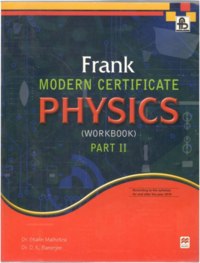## Chapter 2: Light

Exercise 2.1Exercise 2.2Exercise 2.3Exercise

#### Chapter 2: Light Exercise Exercise 2.1 solutions [Page 88]

Exercise 2.1 | Q 1 | Page 88
What do you mean by the term refraction?
Exercise 2.1 | Q 2 | Page 88

State Snell'S Law.

Exercise 2.1 | Q 3 | Page 88
Does reflection also take place with the refraction?
Exercise 2.1 | Q 4 | Page 88
What is the cause of refraction of light?
Exercise 2.1 | Q 5 | Page 88
A ray of light is incident normally on a plane glass slab. What will be the angle of refraction and the angle of deviation for the ray?
Exercise 2.1 | Q 6 | Page 88
Name the phenomenon which enables an empty test tube placed inside water shine like a mirror.
Exercise 2.1 | Q 7 | Page 88
Write the SI unit for refractive index.
Exercise 2.1 | Q 8 | Page 88
What do you mean by the statement that the refractive index of diamond is 2.42?
Exercise 2.1 | Q 9.1 | Page 88

Plot a graph between
Angle of incidence versus angle of reflection,

Exercise 2.1 | Q 9.2 | Page 88

Plot a graph between

Sine of angle of incidence versus sine of angle of refraction,

Exercise 2.1 | Q 9.3 | Page 88

Plot a graph between

Angle of incidence versus angle of refraction.

Exercise 2.1 | Q 10 | Page 88
How is the refractive index of a medium related to the speed of light in it?
Exercise 2.1 | Q 11 | Page 88
For which colour of white light, is the refractive index of a transparent medium the least?
Exercise 2.1 | Q 12 | Page 88
For which colour of white light, is the refractive index of glass the most?
Exercise 2.1 | Q 13 | Page 88
State the principle of reversibility of light.
Exercise 2.1 | Q 14 | Page 88

The refractive index of water respect to air is 4/3. calculate the refractive index of air with respect to water.

Exercise 2.1 | Q 15 | Page 88
Define absolute refractive index of a medium.
Exercise 2.1 | Q 16 | Page 88

Define the term refractive index of a medium. What do you understand by the statement 'the refractive index of glass is 1.5 for white light'?

Exercise 2.1 | Q 17 | Page 88
Is dispersion same as deviation?
Exercise 2.1 | Q 18 | Page 88
Define angle of deviation.
Exercise 2.1 | Q 19 | Page 88
Name the factors on which the deviation produced by a prism depends.
Exercise 2.1 | Q 20 | Page 88

If a light ray does not undergo, refraction at the boundary between two media, the angle of incidence is:

•  0°

• 45°

•   60°

•   90°

Exercise 2.1 | Q 21 | Page 88

A ray of light passes from air to water. In fig. 39, which of the ray A, B, C and D is the correct refracted ray?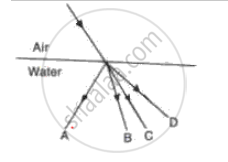Exercise 2.1 | Q 22 | Page 88

In the fig. name the ray which represents the correct path of light while passing through a glass block.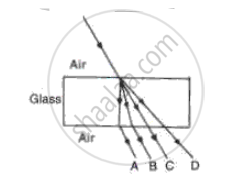Exercise 2.1 | Q 23 | Page 88

Which of the following has the highest refractive index:

• Glass

• Water

• Diamond

• Ruby

#### Chapter 2: Light Exercise Exercise 2.1 solutions [Page 89]

Exercise 2.1 | Q 1 | Page 89

In the fig., PO is a ray of light incident on a rectangular glass block.
(a)   Complete the path of the ray through the block.
(b)  In the diagram, mark the angle of incidence (i) and the angle of refraction (r) at the first interface. How is the refractive index of glass related to the angles I and r?
(c)  Mark angle of emergence by the letter e. How are the angles i and e related?
(d)  Which two rays are parallel to each other? Name them.
(e)  Indicate in the diagram the lateral displacement between the emergent ray and the incident ray.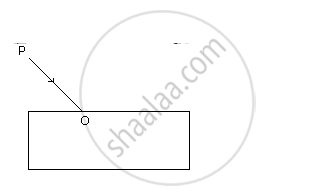Exercise 2.1 | Q 2 | Page 89
State two factors which affect the critical angle for a given pair of media.
Exercise 2.1 | Q 3 | Page 89
The critical angle for glass-air is 450 for the light of yellow colour. State whether it will be less than, equal to, or more than 450 for (i) red light, (ii) blue light?
Exercise 2.1 | Q 4 | Page 89

Fill in the blank to complete the following sentence:
When light travels from a rarer to a denser medium, it bends ...........

Exercise 2.1 | Q 5 | Page 89
Define the terms critical angle and total internal reflection. State two conditions necessary for total internal reflection to occur. Write down the relation for the critical angle in terms of refractive index.
Exercise 2.1 | Q 6 | Page 89
What do you mean by the statement 'the critical angle for diamond is 240'?
Exercise 2.1 | Q 7 | Page 89
Explain the meaning of the term refraction. A ray of monochromatic light is incident from air on a glass slab. Draw a ray diagram indicating the change in its path till it emerges out of the slab.
Exercise 2.1 | Q 8 | Page 89
State two uses of a total reflecting prism as a reflector in place of a plane mirror.
Exercise 2.1 | Q 9 | Page 89

Draw an í- delta graph for a mono chromatic raY through a glass prism of a plane and mark

(i) delta m, the angle of mínimum devíation

(íí) Any two values of i for which value of delta ís same.

#### Chapter 2: Light Exercise Exercise 2.2 solutions [Page 101]

Exercise 2.2 | Q 1 | Page 101
What do you mean by a lens?
Exercise 2.2 | Q 2 | Page 101
State two main differences between a convex and concave lens.
Exercise 2.2 | Q 3.1 | Page 101

Draw neat diagram to show the
Convergent action of a convex lens,

Exercise 2.2 | Q 3.2 | Page 101

Draw neat diagram to show the
Divergent action of concave lens

Exercise 2.2 | Q 4 | Page 101
Define the term focal length of a lens.
Exercise 2.2 | Q 5 | Page 101
Can you guess the focal length of a plane mirror?
Exercise 2.2 | Q 6 | Page 101
Write the SI unit of focal length of a concave lens.
Exercise 2.2 | Q 7 | Page 101
Define the term principle axis of a lens.
Exercise 2.2 | Q 8 | Page 101
What do you understand by focal plane of a lens?
Exercise 2.2 | Q 9 | Page 101
Show by a diagram the refraction of two incident rays parallel to the principle axis passing through a convex lens by treating it as a combination of a glass block and two triangular glass prisms.

#### Chapter 2: Light Exercise Exercise 2.2 solutions [Page 102]

Exercise 2.2 | Q 1 | Page 102
Show by a diagram, the refraction of two incident rays parallel to the principle axis passing through a concave lens by treating it as a combination of a glass block and two triangular glass prisms.
Exercise 2.2 | Q 2.1 | Page 102

State the condition of the following:
A lens has both its focal lengths equal,

Exercise 2.2 | Q 2.2 | Page 102

State the condition of the following:
A ray passes undeviated through the lens.

Exercise 2.2 | Q 3 | Page 102

Fig shows a lens as a combination of a glass block and two prisms.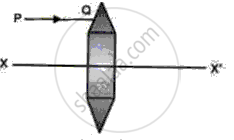(i) Name the lens formed by the combination.

(ii) what is the XX' called?

(iii) Complete the ray diagram and show the path of the incident ray PQ after passing through the lens.

(iv) The final emergent ray will either meet XX' at a point or appear to come from a point on XX'. what is the point called?

Exercise 2.2 | Q 4 | Page 102

Fig shows a lens as a combination of a glass block and two prisms.

(i) Name the lens formed by the combination.

(ii) What is the line XX' called?

(iii) Complete the path of the incident ray PQ after passing through the lens.

(iv) The final emergent ray either meets XX' at a point or appears to come from a point on XX'. Label it as F, What is this point called?

Exercise 2.2 | Q 5 | Page 102
An object of height 4.0 cm is placed at a distance 24 cm in front of a convex lens of focal length 8 cm. Draw a ray diagram to find the position of image. State the characteristics of the image.
Exercise 2.2 | Q 6 | Page 102

Fig shows an object PQ placed on the principle axis of a lens L. The two foci of the kens are F1 and f2. The image formed by the lens is erect, Virtual and dimnished.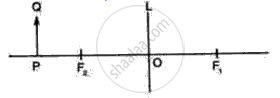(i) Draw the outline ofthe lens L used and Named it.

(ii) Draw a ray of light starting from Q and passing through O. show the same ray after refraction by the lens.

(iii) Draw another ray from Q Which is incident parallel to the principle axis and show how it emerges after refraction from the lens.

(iv) Locate the final image formed.

Exercise 2.2 | Q 7 | Page 102
Distinguish between a real and a virtual image.
Exercise 2.2 | Q 8 | Page 102

Refer to fig

(i) Name the lens L.

(ii) What are the points O, O' called?

(iii) Complete the diagram to form the image of the object AB.

(iv) Write three characteristics of the image.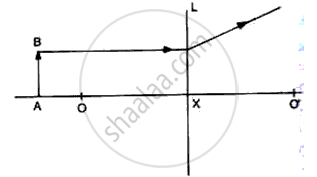#### Chapter 2: Light Exercise Exercise 2.2 solutions [Page 103]

Exercise 2.2 | Q 1 | Page 103
A convex lens produces an image on a screen twice the size of the object. The distance between the object and screen is 45 cm. Find the distance of the lens from the object and its focal length by drawing a ray diagram.
Exercise 2.2 | Q 2.1 | Page 103

Express power of a concave lens of focal length 50 cm with its sign.

Exercise 2.2 | Q 2.2 | Page 103
The power of a lens is +2.0 D. Find its focal length and state what kind of lens it is.

#### Chapter 2: Light Exercise Exercise 2.2 solutions [Page 110]

Exercise 2.2 | Q 1 | Page 110
Will dispersion result when a monochromatic light passes through a prism?

#### Chapter 2: Light Exercise Exercise 2.3 solutions [Page 109]

Exercise 2.3 | Q 1 | Page 109
What do you mean by dispersion?
Exercise 2.3 | Q 2 | Page 109

(i) Longer than 8 x 10 -7 m

(ii) shorter than 4 x 10 -7 m.

Exercise 2.3 | Q 3 | Page 109
What is the velocity of electromagnetic wave?
Exercise 2.3 | Q 4 | Page 109
How does the deviation produced by a triangular prism depend on the colours (or wavelengths) of light incident on it? Which colour deviates the most and which the least?
Exercise 2.3 | Q 5 | Page 109
What do you understand by the term spectrum?
Exercise 2.3 | Q 6 | Page 109

Fig shows a thin beam of white light from a source S striking on one face of prism.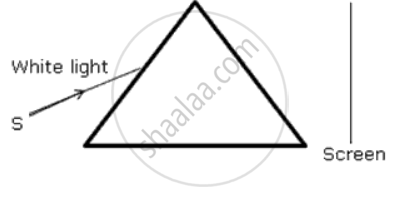(a) Complete the diagram to show the effect of prism on the beam and to show what is seen on the screen.

(b) A slit is placed in between the prism and the sceen to pass only the light of green colour. what will you then oserve on the scren?

(c) What conclusion do you draw from the observation in (b) above?

Exercise 2.3 | Q 7.1 | Page 109

If a monochromatic beam of light undergoes minimum deviation through an equiangular prism, how does the beam pass through the prism, with respect to its base?

Exercise 2.3 | Q 7.2 | Page 109

If white light is used in same way what change is expected in the emergent beam?

Exercise 2.3 | Q 8 | Page 109
Express nanometer (nm) in terms of Angstrom

#### Chapter 2: Light Exercise Exercise 2.3 solutions [Page 110]

Exercise 2.3 | Q 1 | Page 110
What do you mean by spectrum?
Exercise 2.3 | Q 2 | Page 110
What is the cause of dispersion?
Exercise 2.3 | Q 3 | Page 110
Briefly, describe an experiment to show that a prism itself produces no colour.
Exercise 2.3 | Q 4.1 | Page 110

Draw a diagram to show the splitting of white light into its constituent colours.

Exercise 2.3 | Q 4.2 | Page 110

Draw another diagram to show how the colours of spectrum of white light can be combined to give the effect of white light.

Exercise 2.3 | Q 5 | Page 110

Complete the ray diagram given below to show the nature of light produced on the screen.

The completed ray diagram is as shown below :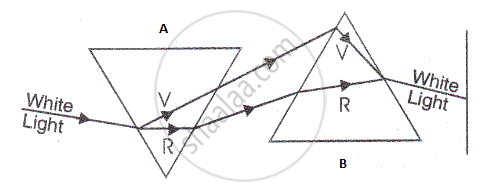Exercise 2.3 | Q 6.1 | Page 110

Name the scientist who discovered
X-rays

Exercise 2.3 | Q 6.2 | Page 110

Name the scientist who discovered Visible light

Exercise 2.3 | Q 6.3 | Page 110

Name the scientist who discovered Microwaves

Exercise 2.3 | Q 6.4 | Page 110

Name the scientist who discovered radio waves

Exercise 2.3 | Q 6.5 | Page 110

Name the scientist who discovered Infra-red waves

Exercise 2.3 | Q 6.6 | Page 110

Name the scientist who discovered Ultraviolet rays

Exercise 2.3 | Q 7 | Page 110
The wavelengths for the light of red and blue colours are roughly 7 X 10-7 m and 4 X 10-7 m respectively. Which colour has the greater speed in vacuum? Which colour has the greater speed in glass?
Exercise 2.3 | Q 8 | Page 110
What is a spectrum? Draw a labeled ray diagram to show the formation of spectrum of white light.

#### Chapter 2: Light Exercise Exercise 2.3 solutions [Page 145]

Exercise 2.3 | Q 1 | Page 145
State any two ways of increasing the frequency of vibration of a stretched string.

#### Chapter 2: Light Exercise Exercise solutions [Page 112]

Exercise | Q 1.1 | Page 112

Make the correct choices in the following items :

A magnified erect image is obtained when an object is positioned in front of a converging lens. The distance of the object from the lens would be

• Greater than three focal lengths

• Equal to two focal lengths

• Between one and two focal lengtghs

• equal to one focal length

• Less than one focal length

Exercise | Q 1.2 | Page 112

Make the rrect choices in the following items :

An object is placed 50 cm from a connverging lens of focal length 30 cm. The image produced would be

• lnverted and same size as the obíect

• lnverted and diminished

• lnverted and magnified

• Erect and diminished

• Erect and magnified

Exercise | Q 1.3 | Page 112

Make the rrect choices in the following items :

ln fig, , a real image of a point objert O is formed, Which of the following statements is true about each of the arrangements?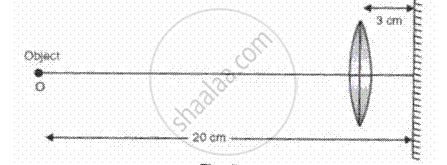(i) The object is at the principle focus of the lens

(ii) The focal length of the lens is 17 cm

(iii) lf the mirror is moved so that it is 6 cm from the lens image will also move

Exercise | Q 1.4 | Page 112

Make the correct choices in the following items :

A lens used as a magnifying glass

(i) ls a diverging lens

(ii) Produces a virtual image

(iii)  ls placed with the object nearer the lens than the principle focus

Exercise | Q 1.5 | Page 112

Make the correct choices in the following items:

lf the image can be focused on a screen it must be

• errect

• virtual

• real

• upright

• coloured

Exercise | Q 1.6 | Page 112

Make the correct choices in the following items :

The image formed by a diverging lens, shown in fig, , is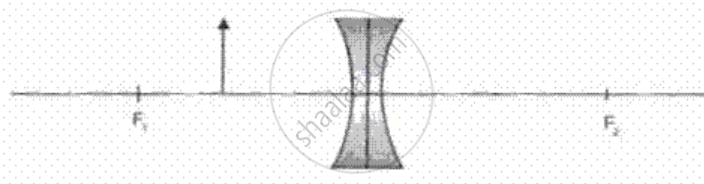• Virtual, erect, magnified

• Virtual, erect, diminished

• Virtual, inverted, magnified

• Real, inverted, diminished

• Real, upright, magnified

Exercise | Q 1.7 | Page 112

Make the correct choices in the following items :

A converging lens B has the same focal length as a converging lens A, but only half the diameter, Both lens are used, separately, to form two images of building on a screen. which one of the follwing statements about the images is correct?

• The image B formed is closer to the lens than the image formed by A.

• The image B formed is bigger than the image formed by A.

• The image B formed is not as sharply focused as the image formed by A

• The image are of the same brightness

• Both the image are real and inverted

#### Chapter 2: Light Exercise Exercise solutions [Page 113]

Exercise | Q 1 | Page 113

A pin 2 cm long is placed 12 cm away from a convex lens at right angles to the principal axis. If the focal length of the lens is 20 cm, by scale drawing find the size of the image and its magnification.

Exercise | Q 2.1 | Page 113

Define the term 'focus' of a lens.

Exercise | Q 2.2 | Page 113

Distinguish between concave and convex lens.

Exercise | Q 3 | Page 113

(a) Draw a sketch to show how a lens is able to produce an image of the sun on a paper screen.
(b)(i)    Would you regard the rays from the sun as being divergent, parallel or convergent?
(ii)    What is the name given to the point where such rays meet after they have passed through the lens?
(iii)    How does the image of the sun sometimes burn a paper screen?

Exercise | Q 4 | Page 113

(a) What type of a lens can be used as a magnifying glass?
(b) Show by a ray diagram the formation of a real image by simple magnifying lens.

#### Chapter 2: Light Exercise Exercise solutions [Page 114]

Exercise | Q 1 | Page 114

Ray diagram for the formation of image by a magnifying glass.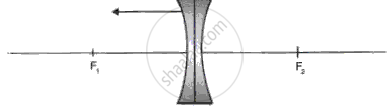Exercise | Q 2.1 | Page 114

What is meant by the power of accommodation produced?

Exercise | Q 2.2 | Page 114

How is accommodation produced?

Exercise | Q 2.3 | Page 114

What type of lenses are used in spectacles worn by an old lady for knitting?

Exercise | Q 3 | Page 114

An object AB is placed in front of a convex lens as shown in fig. 4. Complete the ray diagram and obtain the image formed and state the nature of the image.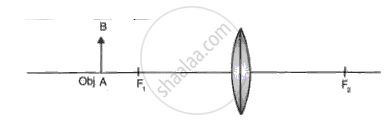Exercise | Q 4 | Page 114

An object is placed in front of a converging lens and in front of a diverging lens as in fig.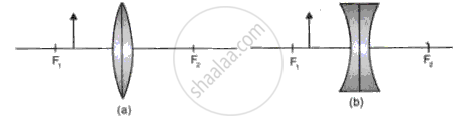(a)Complete the ray diagram to obtain an image.
(b)Compare the nature of image formed by both the lenses in the above case.

Exercise | Q 5.1 | Page 114

In the following diagram ., the object and the image formed by the respective lenses are shown. Complete the ray diagram, and locate the focus. Find the focal length of the lens.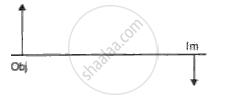Exercise | Q 5.2 | Page 114

In the following diagram  the object and the image formed by the respective lenses are shown. Complete the ray diagram, and locate the focus. Find the focal length of the lens.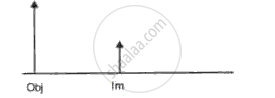Exercise | Q 5.3 | Page 114

In the following diagram , the object and the image formed by the respective lenses are shown. Complete the ray diagram, and locate the focus. Find the focal length of the lens.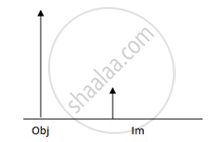Exercise | Q 5.4 | Page 114

In the following diagram , the object and the image formed by the respective lenses are shown. Complete the ray diagram, and locate the focus. Find the focal length of the lens.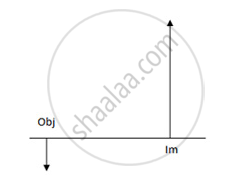#### Chapter 2: Light Exercise Exercise solutions [Page 115]

Exercise | Q 1 | Page 115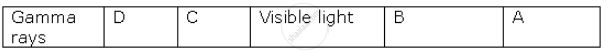The above table shows different parts of the electromagnetic spectrum.
(a)    Identify the parts of the spectrum marked as A, B, C and D.
(b)    Which of the radiations A or B has the higher frequency?
(c)    State two properties which are common to all parts of the electromagnetic spectrum.
(d)    Name one source of each of the radiation of electromagnetic spectrum.
(e)    Name one detector for each of the radiation.
(f)    Name one use of each of the radiation.

Exercise | Q 2.1 | Page 115

Make the correct choice for each of the following:

Which one of the following Fig correctly shows the path of the ray through the glass block?

•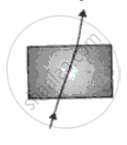•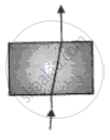•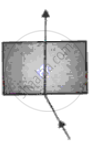•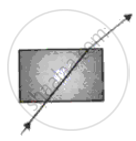•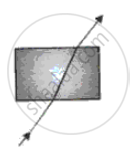Exercise | Q 2.2 | Page 115

Make the correct choices for each of the following :

Total Internal reflection takes place when

(where ∠i = angle of incidence,  ∠r = angle of refraction, ∠C = critical angle)

• Light is going from glass to air and  ∠I < ∠C

• Light is going from air to glss and ∠i < ∠C

• Light is going from glass to air and  ∠i > ∠C

• Light is going from air to glss and ∠i > ∠C

• Light is going from glass to air and  ∠i = ∠r

Exercise | Q 2.3 | Page 115

Make the correct for each of the following :

With reference to the fig, the refractive index of the glass block is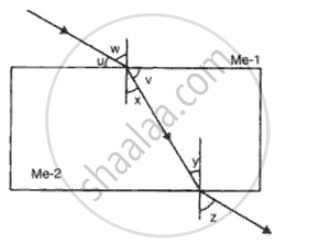• "sin w"/"sin z"

• "sin x"/"sin w"

• "sin u"/"sin v"

• "sin u"/"sin x"

• "sin w"/"sin x"

Exercise | Q 2.4 | Page 115

Make the correct choice for each of the following:

fig illustrates an experiment to determine the refractive index of glass. Which one of the following graphs would give a straight line through the origin?

• ∠i plotted against ∠r.

• Sin i plotted against ∠r.

• ∠i plotted against sin D

• Sin i plotted against Sin r

• 1/(∠"i") " plotted against"  1/(∠"r")

Exercise | Q 2.5 | Page 115

Make the correct choice for each of the following :

Which one of the five diagrams represents the path of a ray of light through a periscope?

•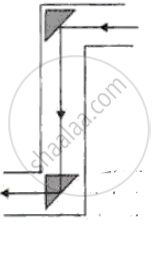•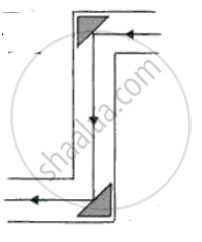•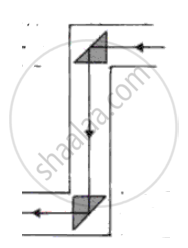•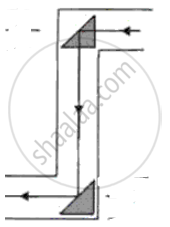•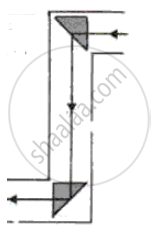#### Chapter 2: Light Exercise Exercise solutions [Page 116]

Exercise | Q 1 | Page 116

Fig. shows two rays of light Op and OQ coming from an object at the bottom of a pond, incident on the water surface.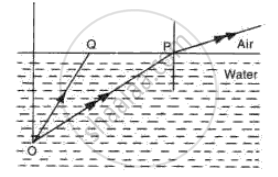(a) Mark on the diagram
(i)     The angle of incidence of ray OP,
(ii)    The angle of refraction of ray Op,
(iii)    The position of image of the object as seen from above.
(iv)    An approximate path of the ray OQ.
(b) Explain, why do the rays of light change directions on passing from water to air.
(c) A fish in water sees everything outside the water by rays of light entering its eye in a small cone of light. Draw a diagram and explain how does this happen.

Exercise | Q 2 | Page 116

(a)A ray of light is incident at 45° on the face of
(i) A rectangular block of glass.
(ii) A 600 glass prism.
(b) Draw a sketch showing how the ray of monochromatic ray of light passes through glass in each case.
(c) With the aid of a diagram, explain how the face of a right angled prism may totally reflect incident on it.
(d) A thick plane mirror produces several faint images in addition to a prominent one. Draw a ray diagram showing how reflection and refraction produce all these images.
(e) Fig. represents a stone S at the bottom of a pond of water. Using the two rays, as shown, complete the ray diagram to show where the image of the stone appears when viewed from E.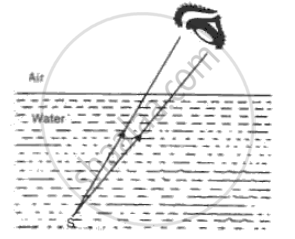(f) What is a''mirage'? Explain with the help of a diagram.
(g) A man observes the bottom of a swimming pool of 3 m depth. If the refractive index of water is 1.3, what is the apparent depth of water?
(h) When a ray of light undergoes refraction through a glass slab and when it emerges it is displaced laterally (Fig). What are the factors on which the lateral displacement depends?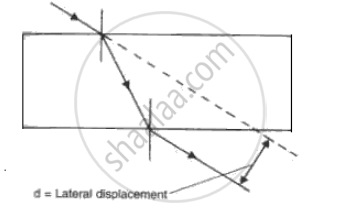(i) Fig. shows three rays of light OA, OB and OC passing from water to air, making angles 490, 410 and 350 with the horizontal surface respectively. Draw an approximate path of the emergent ray for each. (Critical angle of water is 490.)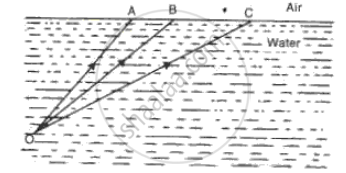#### Chapter 2: Light Exercise Exercise solutions [Page 117]

Exercise | Q 1 | Page 117
Define critical angle and explain total internal reflection. What are the conditions necessary for total internal reflection?
Exercise | Q 2 | Page 117

(a) A ray of monochromatic light enters glass PQRS as shown in the fig. Complete the path of ray till it emerges from the glass. (Critical angle of glass is 420).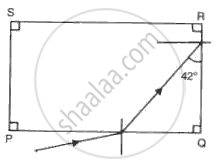(b) Draw diagram of a prism periscope.
(c) What are the advantages of total internal reflecting prism over plane mirror?

Exercise | Q 3 | Page 117

Fig. shows a ray of white light that passes through a prism and produces a spectrum.
(a) Name the phenomenon that is taking place.
(b) What colour would you see at X and Y?
(c) What radiation would you detect above X and below Y?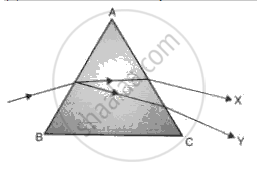#### Chapter 2: Light Exercise Exercise solutions [Page 118]

Exercise | Q 1 | Page 118
Give two properties of ultraviolet radiations which differ from the visible light.
Exercise | Q 2 | Page 118
Mention three properties of infra red radiations similar to the visible light. Give two such properties of infrared radiations which are not true for visible light.
Exercise | Q 3 | Page 118

Two waves A and B have wavelength 0.01 Å and 9000 Å respectively.

Name the two waves. compare the speeds of these waves when they travel in vacuum.

#### Chapter 2: Light Exercise Exercise solutions [Page 119]

Exercise | Q 1 | Page 119

Fig shows part of the arrangement for obtaining pure spectrum.

(a) Complete thediagram and show how to obtain a pure spectrum.

(b) What are the conditions necessary for obtaining a pure spectrum?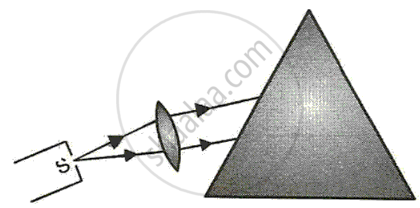Exercise | Q 2 | Page 119
What are ultraviolet radiations? How are they detected? State two properties and one use of ultraviolet radiations.
Exercise | Q 3 | Page 119
Name three properties of ultraviolet radiations which are similar to visible light.
Exercise | Q 4 | Page 119
An electromagnetic wave has a frequency of 500 MHz and a wavelength of 60 cm. Calculate the velocity of the wave. Name the medium through which it is traveling.

## Chapter 2: Light

Exercise 2.1Exercise 2.2Exercise 2.3Exercise

#### Frank Frank Class 10 Physics Part 2## Frank solutions for Class 10 Physics chapter 2 - Light

Frank solutions for Class 10 Physics chapter 2 (Light) include all questions with solution and detail explanation. This will clear students doubts about any question and improve application skills while preparing for board exams. The detailed, step-by-step solutions will help you understand the concepts better and clear your confusions, if any. Shaalaa.com has the CISCE Frank Class 10 Physics Part 2 solutions in a manner that help students grasp basic concepts better and faster.

Further, we at Shaalaa.com provide such solutions so that students can prepare for written exams. Frank textbook solutions can be a core help for self-study and acts as a perfect self-help guidance for students.

Concepts covered in Class 10 Physics chapter 2 Light are Refraction of Light Through a Glass Block and a Triangular Prism, Total Internal Reflection, Total Internal Reflection - Critical Angle, Applications of Refraction of Light, Refraction of Light Through Plane Surface, Electromagnetic Spectrum, Some Natural Phenomena Due to Sunlight, Using a Triangular Prism to Produce a Visible Spectrum from White Light, Concept of Spectrum, Image Formation by Lenses - Convex Lens, Image Formation in Lenses Using Ray Diagrams - Concave Lens, Concept of Magnifying Glass, Types of Lenses (Converging and Diverging),, Refraction by a Lens, Power of a Lens, Concept Refraction of Light Through a Lense.

Using Frank Class 10 solutions Light exercise by students are an easy way to prepare for the exams, as they involve solutions arranged chapter-wise also page wise. The questions involved in Frank Solutions are important questions that can be asked in the final exam. Maximum students of CISCE Class 10 prefer Frank Textbook Solutions to score more in exam.

Get the free view of chapter 2 Light Class 10 extra questions for Physics and can use Shaalaa.com to keep it handy for your exam preparation

S